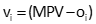## 1. General

The most probable value (MPV) estimation of an observation set is the one which that minimizes the sum of the squares of the residuals, Equations C-1 and C-2.Equation C-1Equation C-2 v: Residual o: Observation

Combining Equations C-1 and C-2 gives Equation C-3; its only unknown is the MPV. To minimize a function, its first derivative is set equal to zero, Equation C-4, and solved for the unknown.Equation C-3Equation C-4

Because the derivative is set to zero, we will refer to this as the Direct Minimization method.